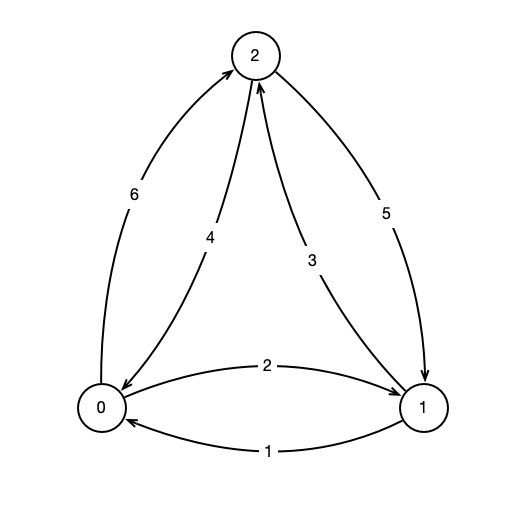Lab 02: Computing Shortucts

A NetworkNetwork

• nodes and edges between nodes
• nodes labeled $0, 1, \ldots, n$
• directed edges $(i, j)$ from $i$ to $j$ for each $i \neq j$
• edges $(i, j)$ have associated weight, $w(i, j) \geq 0$
• weight indicates cost or distance to move from $i$ to $j$

ShortcutsWhat is cheapest path from 0 to 2?

A Problem

Given a network as above, for all $i \neq j$, find cheapest path of length (at most) 2 from $i$ to $j$

• weight of a path is sum of weight of edges
• convention: $w(i, i) = 0$
• a shortcut from $i$ to $j$ is a path $i \to k \to j$ where $w(i, k) + w(k, j) < w(i, j)$

Shortcut DistancesRepresenting Input• $D = \left( \begin{array}{ccc} 0 & 2 & 6\\ 1 & 0 & 3\\ 4 & 5 & 0 \end{array} \right)$

Computing Output

• $D = (d_{ij})$
• Output $R = (r_{ij})$
• $r_{ij}$ = shortcut distance from $i$ to $j$
• computed by $$r_{ij} = \min_k d_{ik} + d_{kj}$$

Example

• $D = \left( \begin{array}{ccc} 0 & 2 & 6\\ 1 & 0 & 3\\ 4 & 5 & 0 \end{array} \right)$
• $R = \left( \begin{array}{ccc} 0 & 2 & 5\\ 1 & 0 & 3\\ 4 & 5 & 0 \end{array} \right).$

In Code

• Create a SquareMatrix object
• SquareMatrix stores a 2d array of floats called matrix
• matrix[i][j] stores $$w(i, j)$$